### Home > CC4 > Chapter 7 > Lesson 7.2.2 > Problem7-99

7-99.
1. In this lesson, you discovered that the midsegment of a triangle is not only parallel to one of the sides, but also half that side’s length. But what about the midsegment of a trapezoid? 7-99 HW eTool (Desmos). Homework Help ✎

2.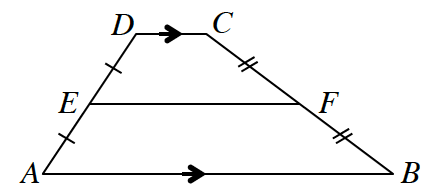The diagram at right shows a midsegment of a trapezoid. That is, EF is a midsegment because points E and F are both midpoints of the non-base sides of trapezoid ABCD.

1. If A(0, 0), B(9, 0), C(5, 6), and D(2, 6), find the coordinates of points E and F. Then compare the lengths of the bases (AB and CD) with the length of the midsegment EF. What is the relationship among the segments?

2. Verify that the relationship you observed in part (a) holds if A(–4, 0), B(2, 0), C(0, 2), and D(–2, 2).

3. Write a conjecture about the midsegment of a trapezoid.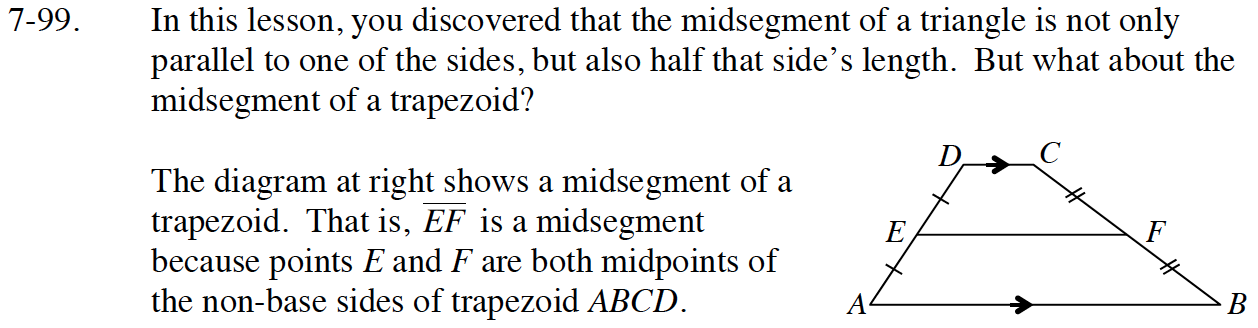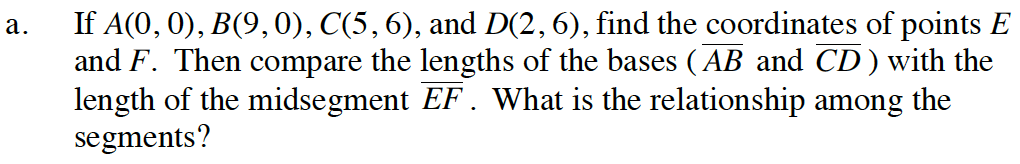How do you find the lengths from coordinates?
Think of how the answers could be related.

It seems to be the average of AB and CD.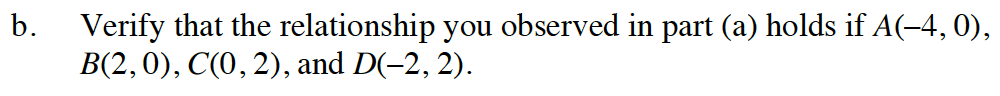Use the same method you used to solve part (a).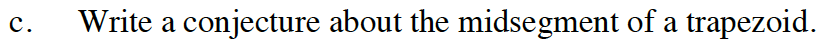The midsegment of a trapezoid is parallel to the bases and has a length that is the average of the lengths of the bases.

Use the eTool below to help solve the problem.
Click the link at the right to view full version of the eTool: Int1 7-99 HW eTool.